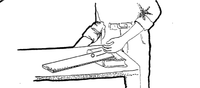# 11. EASY AS 1-2-3

from Come Fly With Me – Exploring Science through aviation and aerospace concepts.

SUBJECT: Science
GROUP SIZE: Large or Small
TIME: 60 minute period
TYPE OF ACTIVITY: Student Investigation or Teacher Demo
TEACHING STRATEGY: Guided Discovery
CONCEPTS: Newton’s Three Laws – Inertia, Acceleration, Action/Reaction
SKILLS: Observation Collecting and Interpreting Data
Objective: To learn and experience examples of Newton’s three laws of motion.

Materials: Marble or other small sphere; two meter sticks taped together in a “v” shape; three spring scales; plastic cup; index card; coin; stop watch.

Teacher Background Information: An understanding of the motion of planes and rockets requires a general knowledge of Newton’s three laws. The following activities are specific to each of the laws and will help the student see relationships among all three.
Procedure:

Newton’s First Law… Generally stated as, “Things in motion tend to remain in motion, things at rest tend to remain at rest.” The tendency to stay at rest or, once moving, in motion is called INERTIA. Things move or stop only if acted upon by another force. To demonstrate:

1. Set a container on a table.
2. Place a card on top of the container and place a quarter on top of the card.
3. Flick the card away with your finger.
4. Did the coin have inertia? What force acted on the card to set it in motion? What force acted on the coin after step 3? (If the card is flipped out of the way fast enough the coin will remain over the container and fall into it.)

Newton’s Second Law… “Acceleration of an object increases as the force causing acceleration increases.”

1. Prepare a ramp for a marble or other sphere about 1 meter long.
2. Lift one end of the ramp and place a pencil under it.
3. Roll a marble down the ramp and time the roll from one end to the other.
4. Lift the ramp higher. Place a book under the lifted end and repeat step 1.
5. Start the marble on a ramp with one end pencil high. As the marble starts out, lift the end of the ramp higher. What was the effect on the speed of the marble?
6. Compare the results of the first two experiments. What was the result? What was the force that was acting on the marble? How was it increased? What was the effect of this increase?

Newton’s Third law… “For every action there is an opposite and equal reaction.”

1. Take two spring scales and hook them together.
2. Have two students pull on the scales in opposite directions. Record the readings from both scales.
3. Place a third scale between the other two. Record the readings from all three. What were the differences in the readings for the two scales? When three scales were used? (The readings should all be the same in each experiment.)

Extensions: Have the students apply the principles of Newton’s laws to the description of

1. A plane taking off and climbing to 2000 feet while accelerating to 120 miles per hour.
2. A three stage rocket launched into orbit around the Earth then sent on to the moon. Which of Newton’s laws are being applied in each case and at what point are they being applied?

This site uses Akismet to reduce spam. Learn how your comment data is processed.